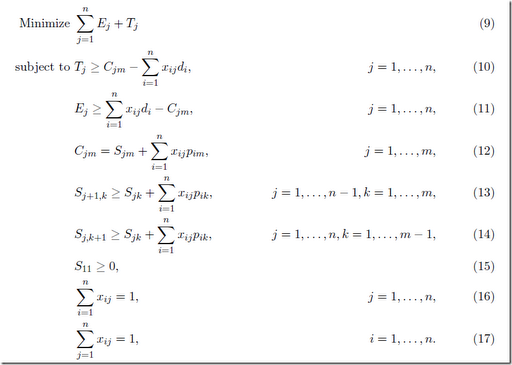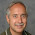## Friday, May 18, 2012

### Model improvements

Looking at the models used in the paper http://www.ime.usp.br/~egbirgin/publications/rob.pdf I noticed that a few standard “improvements” could be introduced. Here is an example:First equations (13) and (14) have the same RHS. Hence it makes sense to introduce a new variable for this.

Secondly, when we look at the AMPL models provided by the authors, we see:

 R1 {j in 1..n}:     T[j] >=   ( S[j,m] + sum{i in 1..n} ( x[i,j] * p[i,m] ) )             - sum {i in 1..n} ( x[i,j] * d[i] ); R2 {j in 1..n}:     E[j] >= - ( S[j,m] + sum{i in 1..n} ( x[i,j] * p[i,m] ) )             + sum {i in 1..n} ( x[i,j] * d[i] );

I.e. they substituted out C[j,m]. I would probably leave that as it was formulated in the paper.

Now, do these improvements really work? As is sometimes the case: the results on a single instance can be misleading. So here were my experiments:

 old version new version size rows 335 500 cols 405 570 nz 5635 3865 gurobi iterations 1060935 453173 nodes 31220 5971 time 60 38 cbc iterations 4589173 4790425 nodes 36154 49851 time 395 478

We see that my version has fewer nonzero elements at the expense of more variables and equations. But not with every solver (or with every instance) this pays off. CBC actually becomes slower. Most likely this can be explained with the simple argument that the solver follows a different path. To really assert that a formulation is better one really needs to run a large number of models and see how they behave on average.

Note: the reformulated model (and the computational results) already included the suggestion in the comment from Paul.

#### 1 comment:

1.Your comment about (13) and (14) would also apply to (10) and (11), give or take a minus sign. That would further increase size and further reduce nonzeros. I wonder what impact it would have -- more in the same direction (Gurobi even better, CBC even worse), or not?